# Intermediate Algebra Tutorial 20

Intermediate Algebra
Tutorial 20: Solving Systems of Linear Equations
in Three VariablesAnswer/Discussion to 1a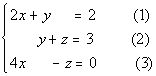Note that the numbers in (  ) are equation numbers.  They will be used throughout the problems for reference purposes.

Step 1: Simplify and put both equations in the form Ax + By + Cz = D if needed.

No simplification needed here.  Let's go on to the next step.

Step 2: Choose to eliminate any one of the variables from any pair of equations.

Note that each equation is missing a variable.  For example, equation (1) is missing z.  We can use this to our advantage.  We can use the equation in it's original form as an equation with a variable eliminated.

I choose to eliminate z.

Since z is already eliminated from the first equation we will use that first equation in its original form for this step: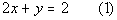*z is already eliminated from eq. (1)

Now we can't just stop here for of two reasons.  First, we would be stuck because we have one equation and two unknowns.  Second, when we solve a system it has to be a solution of ALL equations involved and we have not incorporated the third equation yet.  Let's do that now.

Step 3:  Eliminate the SAME variable chosen in step 2 from any other pair of equations, creating a system of two equations and 2 unknowns.

We are still going after eliminating z, this time I want to use the second and the third equations.

Since the z terms of the second and third equations are already opposites,  we can go right into adding them.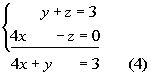*z's have opposite coefficients

*z's dropped out

Step 4:  Solve the remaining system found in step 2 and 3, just as discussed in Tutorial 19: Solving a System of Linear Equations in Two Variables.

Putting the two equations that we have found together we now have a system of two equations and two unknowns, which we can solve just like the ones shown in tutorial 19 (Solving a System of Linear Equations in Two variables) .  You can use either elimination or substitution.  I'm going to go ahead and stick with the elimination method to complete this.

Let's first put those equations together: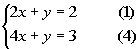*Put equations found in steps 2 and 3
together into one system

I'm going to choose y to eliminate.  I can either multiply the first equation by -1 or the second, either way will create opposites in front of the y terms.

Multiplying equation (4) by -1 and then adding the equations together we get: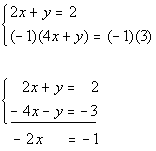*Mult. eq. (4) by -1

*y's have opposite coefficients

*y's dropped out

Solving for x we get: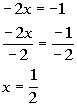*Inverse of mult. by -2 is div. by -2

If we go back one step to the system that had two equations and two variables and plug in ½ for x in equation (1) we  would get: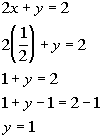*Eq. (1)

*Plug in 1/2 for x

*Inverse of add 1 is sub. 1

Step 5:  Solve for the third variable.

Now we need to go back to the original system and pick any equation to plug in the two known variables and solve for our last variable .

I choose equation (2) to plug in 1 for y that we found: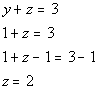*Eq. (2)
*Plug in 1 for
*Inverse of add 1 is sub. 1

Step 6:  Check.

You will find that if you plug the ordered triple (1/2, 1, 2) into ALL THREE equations of the original system, that this is a solution to ALL THREE of them.

(1/2, 1, 2) is a solution to our system.Answer/Discussion to 1b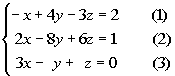Note that the numbers in (  ) are equation numbers.  They will be used throughout the problems for reference purposes.

Step 1: Simplify and put both equations in the form Ax + By + Cz = D if needed.

No simplification needed here.  Let's go on to the next step.

Step 2: Choose to eliminate any one of the variables from any pair of equations.

Let's start by picking our first variable to eliminate.  I'm going to pick z to eliminate.  We need to do this with ANY pair of equations.

Let's first eliminate z using the first and second equations.  This process is identical to how we approached it with the systems found in Tutorial 19: Solving a System of Linear Equations in Two variables .  If I multiply 2 times the first equation, then the z terms will be opposites of each other and ultimately drop out.

Multiplying 2 times the first equation and then adding that to the second equation we get: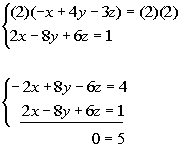*Mult. eq (1) by 2

*z's have opposite coefficients

*All three variables drop out AND
we have a FALSE statement

Wow, that was quick.  All of our variables dropped out and we ended up with a FALSE statement.  You know what that means?  No solution.

Step 3:  Eliminate the SAME variable chosen in step 2 from any other pair of equations, creating a system of two equations and 2 unknowns.

Since we have no solution, there is no value to be found here.

Step 4:  Solve the remaining system found in step 2 and 3, just as discussed in Tutorial 19: Solving a System of Linear Equations in Two Variables.

Since we have no solution, there is no value to be found here.

Step 5:  Solve for the third variable.

Since we have no solution, there is no value to be found here.

Step 6:  Check.

No ordered triples to check.